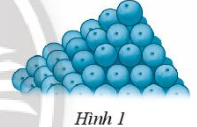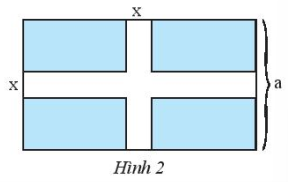## Solving SBT Lesson 1: Algebraic Expressions (C7 SBT Math 7 Horizons) – Math Book

Solving SBT Lesson 1: Algebraic Expressions (C7 SBT Math 7 Horizons)
————-

### Solution 1 page 25 SBT Math 7 Creative horizon episode 2 – CTST

Write a numerical expression representing the area of ​​a parallelogram whose base length is 6 cm and height is 5 cm.

Detailed instructions for solving Lesson 1

Solution method

Apply the formulas for the area of ​​known planes to write the expression.

Detailed explanation

The area of ​​a parallelogram is equal to the product of its base and height.

Therefore, the area of ​​the parallelogram is $$5.6$$ cm2.

–>

— *****

### Solve problem 2 page 25 SBT Math 7 Creative horizon episode 2 – CTST

Write an algebraic expression representing the number of oranges arranged in the top 4 layers of the block in Figure 1.Detailed instructions for solving Lesson 2

Solution method

We see the number of oranges in each layer arranged in a square. So we just need to count the number of oranges on the front of the cube and use the formula to calculate the area of ​​the square.

Detailed explanation

We see the number of oranges in each layer arranged in a square.

Row 1 has the number of results $${1^2}$$; row 2 has the result $${2^2}$$; row 3 has the number of results $${3^2}$$; row 4 has a result of $${4^2}$$

The algebraic expression for the number of oranges arranged in the top 4 layers of the block is $${1^2} + {2^2} + {3^2} + {4^2}$$.

–>

— *****

### Solution 3 page 25 SBT Math 7 Creative horizon episode 2 – CTST

Write an algebraic expression for the area of ​​a rhombus whose first diagonal is 4 cm longer than the second.

Detailed instructions for solving Lesson 3

Solution method

Step 1: Set the hide and its condition.

Step 2: Represent other quantities according to the set implicits.

Step 3: Represent the relationship between the implicit quantities by mathematical operations.

Detailed explanation

Let the length of the first diagonal be $$x\,\left( {x > 0} \right)$$ cm.

The length of the second diagonal is $$x – 4$$ cm.

The algebraic expression for the area of ​​the rhombus is $$\frac{1}{2}.x.\left( {x – 4} \right) = \frac{1}{2}{x^2 } – 2x$$ cm2.

–>

— *****

### Solution 4 page 25 SBT Math 7 Creative horizon episode 2 – CTST

Write an algebraic expression that represents the volume of a rectangular box whose length is 6 cm more than its width and 3 cm more than its height.

Detailed instructions for solving Lesson 4

Solution method

Step 1: Set the hide and its condition.

Step 2: Represent other quantities according to the set implicits.

Step 3: Represent the relationship between the implicit quantities by mathematical operations.

Detailed explanation

Let the length of the rectangle be $$x\,\left( {x > 0} \right)$$ cm.

The width of the rectangle is $$x – 6$$ cm

The height of the rectangle is $$x – 3$$ cm.

The algebraic expression for the volume of a rectangular box is $$x\left( {x – 6} \right)\left( {x – 3} \right) = {x^3} – 3{x^ 2} – 6x + 18$$ cm3.

–>

— *****

### Solve problem 5 pages 25 SBT Math 7 Creative horizon episode 2 – CTST

Write an algebraic expression that represents:

a) Sum of $$3{x^2} + 1$$ and $$5\left( {y – 2} \right)$$;

b) Sum of the squares of $$a + 2$$ and $$b – 1$$.

Detailed instructions for solving Lesson 5

Solution method

Express algebraic expressions by mathematical operations required by the problem.

Detailed explanation

a) The sum of $$3{x^2} + 1$$ and $$5\left( {y – 2} \right)$$ is $$\left( {3{x^2} + 1}$$ right) + 5\left( {y – 2} \right)\)

b) The sum of the squares of $$a + 2$$ and $$b – 1$$ is $${\left( {a + 2} \right)^2} + {\left( {b – 1} \right)^2}$$.

–>

— *****

### Solution 6 page 25 SBT Math 7 Creative horizon episode 2 – CTST

The temperature in a town in the morning is x $$^\circ C$$, at noon it increases by y $$^\circ C$$, and in the evening it decreases by t $$^\circ C$$so with noon. Write an expression for the temperature at night. Calculate the temperature in the evening when $$x = 25;\,y = 5;\,t = 7$$.

Detailed instructions for solving lesson 6

Solution method

Step 1: Represent the relationship between the quantities in the implicit by mathematical operations.

Step 2: Substitute the values ​​of the variable to calculate the temperature in the evening.

Detailed explanation

The expression for the evening temperature is: $$x + y – t$$.

The temperature at night when $$x = 25;\,y = 5;\,t = 7$$ is $$25 + 5 – 7 = 23$$$$^\circ C$$.

–>

— *****

### Solution 7 page 25 SBT Math 7 Creative horizon episode 2 – CTST

Simplify the following expressions:

a) $$4\left( {2y – 3x} \right) – 3\left( {x – 2y} \right)$$

b) $${x^2} + 5y – 2y – 7{x^2}$$

Detailed instructions for solving lesson 7

Solution method

Use the rules of punctuation, breaking brackets learned to shorten expressions.

Detailed explanation

a) $$4\left( {2y – 3x} \right) – 3\left( {x – 2y} \right) = 8y – 12x – 3x + 6y = \left( {8y + 6y} \right) + \left( { – 12x – 3x} \right) = 14y – 15x$$

b) $${x^2} + 5y – 2y – 7{x^2} = \left( {{x^2} – 7{x^2}} \right) + \left( {5y – 2y} \right) = – 6{x^2} + 3y$$

–>

— *****

### Solve problem 8 page 26 SBT Math 7 Creative horizon episode 2 – CTST

A rectangular garden has a width of a (m), length 6m more than width. The walkway is x (m) wide (see Figure 2). Write an expression that represents the area of ​​the remainder of the garden. Calculate the area when a = 30 m, x = 1m.Detailed instructions for solving Lesson 8

Solution method

Step 1: Set the hide and its condition.

Step 2: Represent other quantities according to the set implicits.

Step 3: Represent the relationship between the implicit quantities by mathematical operations.

Detailed explanation

Let the width of the rectangle be $$a$$ (m) $$\left( {a > 0} \right)$$

According to the problem, we have: length is 6m more than width, so length of rectangle is $$a + 6$$(m)

The path area is $$far + x.\left( {a + 6} \right) – {x^2}$$

The area of ​​the rectangle is $$a.\left( {a + 6} \right)$$

The remaining area is $$a.\left( {a – 6} \right) – \left[ {x.a + x.\left( {a + 6} \right) – {x^2}} \right]$$.

Area left when $$a = 30$$ m, $$x = 1$$m is $$a.\left( {a + 6} \right) – \left)[ {x.a + x.\left( {a + 6} \right) – {x^2}} \right] = 30.\left( {30 + 6} \right) – \left[ {1.30 + 1.\left( {30 + 6} \right) – {1^2}} \right] = 1015$$ m2

–>

— *****

### Solve lesson 9 page 26 SBT Math 7 Creative horizon episode 2 – CTST

Every morning you practice walking combined with running. The walking speed is 4 km/h and the running speed is 8 km/h.

a) Write an expression representing the distance that Thu walked x hours and ran y hours.

b) Calculate the distance when $$x = 15$$ minutes and $$y = 30$$ minutes.

Detailed instructions for solving Lesson 9

Solution method

Step 1: Express other quantities in terms of the given unknowns.

Step 2: Represent the relationship between the quantities in the implicit by mathematical operations.

Detailed explanation

a) The expression that represents the distance that Thu has walked x hours and run y is now $$4.x + 8.y$$.

b) Convert $$x = 15$$ minutes $$= 0.25$$ hours and $$y = 30$$ minutes $$= 0.5$$hours.

The distance that you travel is: $$4.0.25 + 8.0.5 = 5$$km.

–>

— *****